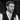# What is a Higher-Order Function?

Nick Scialli March 07, 2020🚀🚀 6 minute read

If you're enjoying this blog, please consider one or both of the following:

One term you might hear in the JavaScript world is “higher-order function.” Today, we’ll explore what it means to be a higher-order function and look at some examples in JavaScript!

# A Definition

By definition, a higher-order function is a function that either takes a function as an argument or returns a function.

If you’re not familiar with treating functions as first class objects , you might be surprised that this is possible. But it is—and it’s extremely powerful!

# Some Simple Examples

Let’s look at a couple simple examples: one for a function that takes a function as an argument and another that returns a function.

## Taking a function as an argument

Let’s create a relatively useless function called `evaluatesToFive` that takes two arguments: the first argument will be a number and the second argument will be a function. Inside our `evaluatesToFive` function, we’ll check if passing the number to the function evaluates to five.

``````function evaluatesToFive(num, fn) {
return fn(num) === 5;
}``````

We can check it out in action:

``````function divideByTwo(num) {
return num / 2;
}

evaluatesToFive(10, divideByTwo);
// true

evaluatesToFive(20, divideByTwo);
// false``````

A bit useless, but it’s cool we can do this!

## Returning a function

In our next example, we’re going to create a function that returns a function. Our function-creating function will be called `multiplyBy`. It will take a number as an argument and return a new function that multiplies its input by that number.

``````function multiplyBy(num1) {
return function(num2) {
return num1 * num2;
};
}``````

Now, we’ll see it in use by creating a couple multiplier functions:

``````const multiplyByThree = multiplyBy(3);
const multiplyByFive = multiplyBy(5);

multipyByThree(10); // 30

multiplyByFive(10); // 50``````

Again, not super useful in its current form but pretty cool regardless.

# A More Complex and Potentially Useful Example

A more useful example of higher-order functions in action is an object validator. The basic idea is a function that takes an object as an argument and then any number of functions that must evaluate to `true` for the object to be considered valid.

In this example, we’ll be handling a `newUser` object and trying to determine if we should allow them to sign up for our application. The user must meet the following criteria:

• Must be at least 18 years old
• Password must be at least 8 characters long

An ideal `newUser` object would look something like this:

``````const newUser = {
age: 24,
agreeToTerms: true,
};``````

Based on this knowledge, we can create some test functions that return `true` when our desired conditions are met and `false` otherwise.

``````function oldEnough(user) {
return user.age >= 18;
}

}

function agreeToTerms(user) {
return user.agreeToTerms === true;
}``````

Now, we can create a function that takes any number of arguments. The first argument will be the object we’re trying to validate and the rest of the arguments will be test functions that will be used to test our object.

``````function validate(obj, ...tests) {
for (let i = 0; i < tests.length; i++) {
if (tests[i](obj) === false) {
return false;
}
}
return true;
}``````

So what exactly is going on here? Here’s a walkthrought:

1. We specify that our first argument to the function is an object (`obj`). Then, we use the rest operator (`...tests`) to say that any additional arguments will be in the `tests` array.
2. We use a `for` loop to iterate through our `tests` array, which is an array of functions (this is the higher-order part!).
3. We pass `obj` to each item in the `tests` array. If that function evaluates to `false`, we know `obj` is invalid and immediately return `false`.
4. If we get through the entire `tests` array without returning `false`, our object is valid and we return `true`.

## Seeing it in action

Now we put our validate higher-order function to use by validating a couple potential new user objects:

``````const newUser1 = {
age: 40,
agreeToTerms: true,
};

// true

const newUser2 = {
age: 40,
agreeToTerms: true,
};

// false``````

And there we have it! `newUser1` is correctly considered to be valid but `newUser2` is detected to be invalid since its `password` is too short.

## A potential improvement: a validator-creating function

Bonus points: if we’re applying our `validate` function to multiple users, it’s probably a better idea to not have to repeatedly specify the same tests over and over again. Instead, we can have a `createValidator` function that returns an object validator. In this case, we’ll create a `userValidator` that applies the same test functions to any user we try to validate.

``````function createValidator(...tests) {
return function(obj) {
for (let i = 0; i < tests.length; i++) {
if (tests[i](obj) === false) {
return false;
}
}
return true;
};
}``````

Let’s see how this gives us a more consistent interface as we validate our `newUser1` and `newUser2` objects again:

``````const userValidator = createValidator(
oldEnough,
agreeToTerms
);

userValidator(newUser1); // true
userValidator(newUser2); // false``````

Awesome! By employing our `createValidator` higher-order function, there is no way we can accidentally use different validation criteria for our different objects.

ReferencesNick Scialli is a software engineer at the U.S. Digital Service.

### Subscribe to the mailing list!

If you like what I post here, please sign up to get updates and code insights in your inbox. I won't spam you and you can unsubscribe any time!